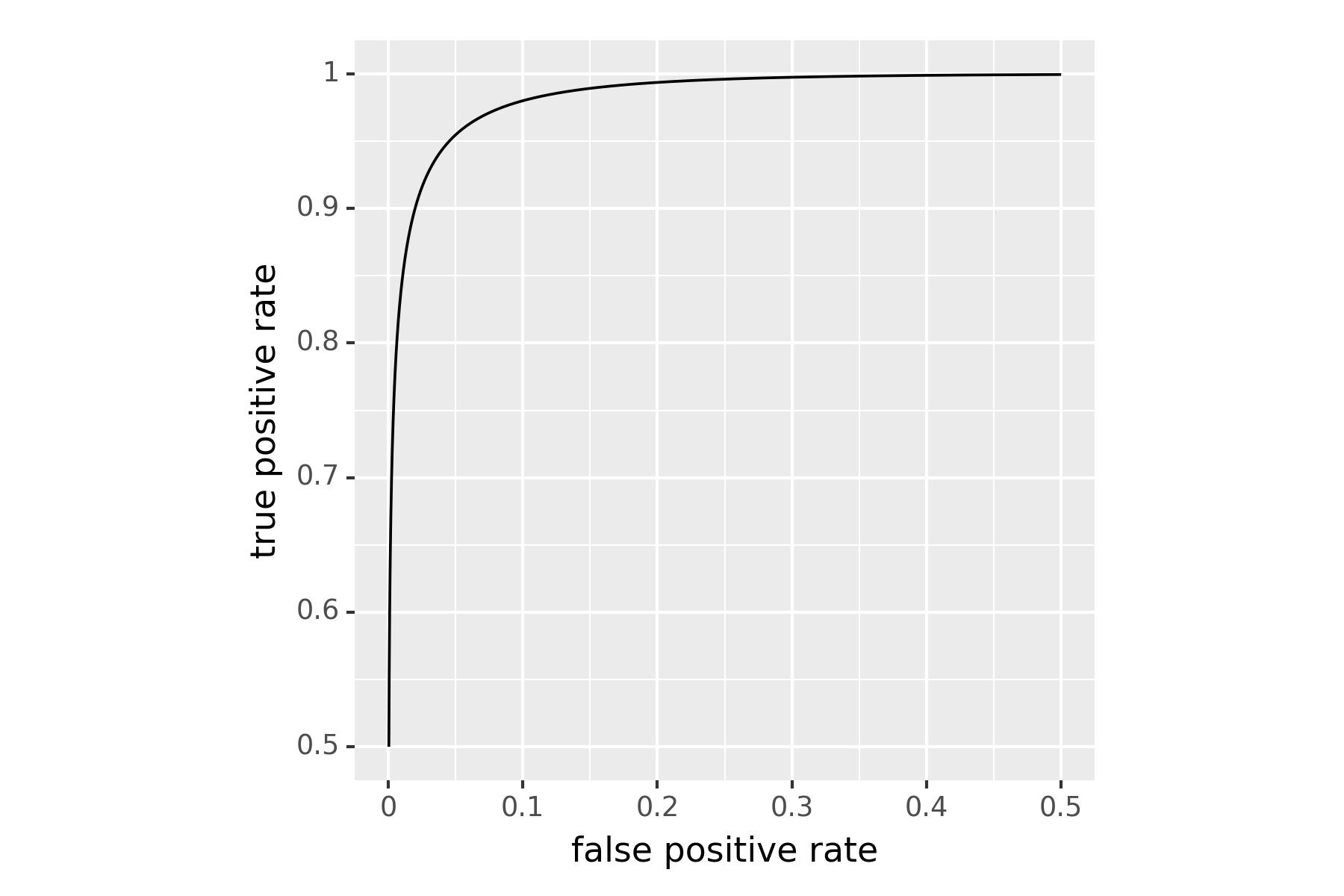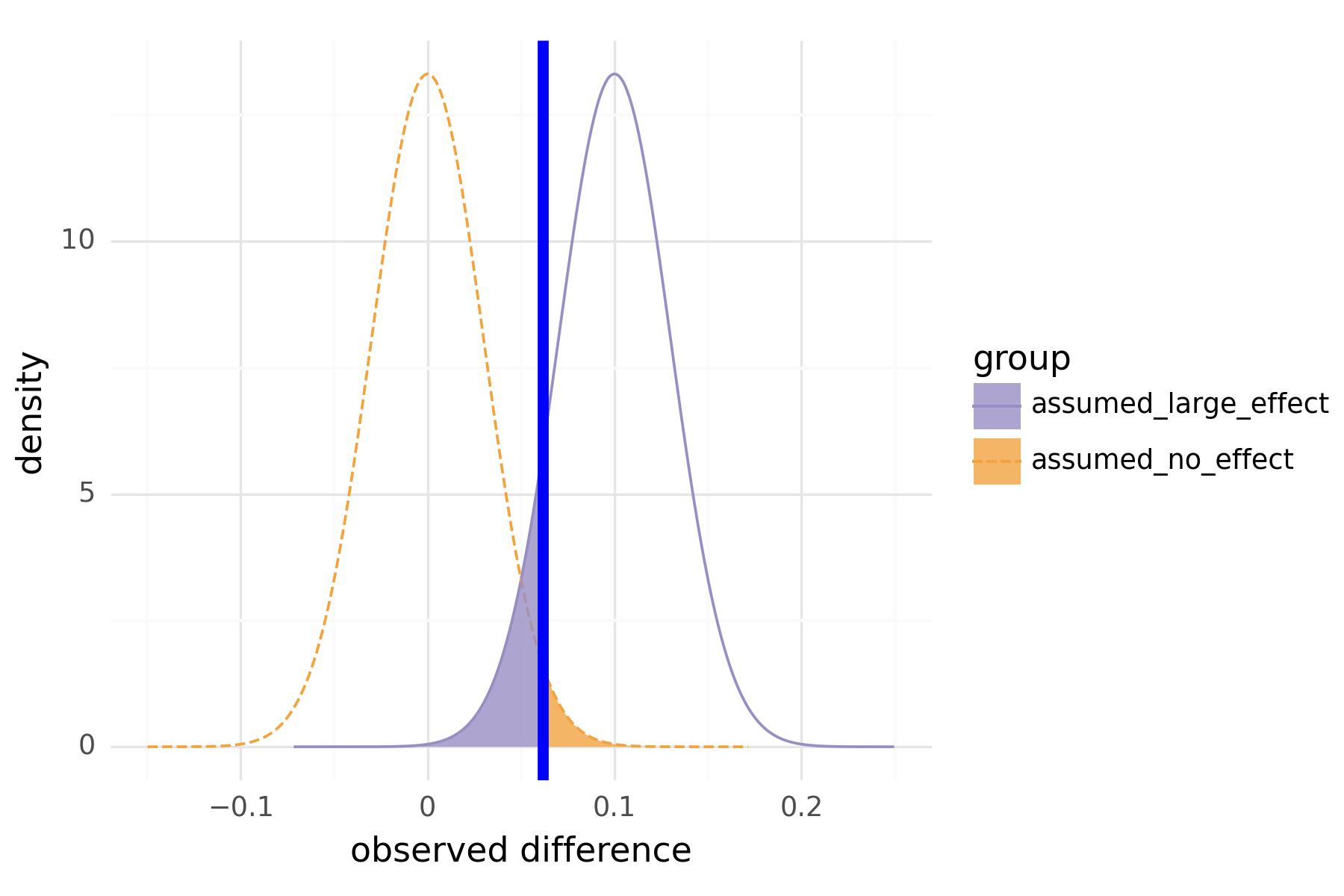# Unrolling the ROC Curve

Most readers of this blog are likely familiar with the use of the ROC (Receiver Operating Characteristic) curve (or, at least, the area under that curve) for evaluating the quality of binary decision processes. One example of such a process is a binary classification model; another example is an A/B test. Yet another example is interpreting a radar signal to detect the presence of objects—the application area from which ROC curves originate.1

All these processes have two components:

1. a signal: the probability or score returned by the classifier; the observed difference between A and B; the electrical signal produced by the radar receiver.
2. a threshold: if the signal is greater than the threshold, then make one decision (we’ll call this “the positive decision”, or “yes”), otherwise, make the other (“no”).

The goal is to make the right decision as often as possible: to say “yes” almost always when in a positive situation (to have a high true positive rate), and to say “yes” only rarely when in a negative situation (to have a low false positive rate). The ROC curve for a given decision signal process traces out the tradeoff between true positive rate and false positive rate as the decision threshold changes. Thus, the ROC shows how well using this decision signal will help you meet your goal.Figure 1: The ROC curve shows the tradeoff between false positive rate and true positive rate for a given decision process.

In this post, we’ll dive a little deeper into the relationship between the ROC curve and decision thresholds. Let’s start with a graph from John Mount’s previous article, on selecting thresholds for A/B tests.Figure 2: Distributions of `mean(B) - mean(A)` under the assumptions of no effect (left) and large effect (right). The blue line is the decision threshold for this experiment.

This graph shows the (ideal) distributions of the two hypotheses for an A/B test of size `2*n`; the left curve is the distribution of `mean(B) - mean(A)` under the null (or negative) situation where B is equivalent to A. The right curve is the distribution of `mean(B) - mean(A)` under the alternate (or positive) situation where B is better than A by an amount `r`=0.1. The horizontal line is the decision threshold. When you run your test, if the final observed `mean(B) - mean(A)` is greater than the threshold, your decision is “yes, the difference I saw is enough for me to say that B is better than A.” The analogous graph for classifier models is the double density graph, where the two distributions would be the distributions of the model score on negative and positive instances, respectively.

The shaded areas in the graph represent errors. The right tail of the negative distribution represents the false positive rate (the probability you’ll decide “yes” when the answer should have been “no”). The left tail of the positive distribution represents the false negative rate (the probability you’ll decide “no” when the answer should have been “yes”). The false negative rate is `1 - true_positive_rate`, since the true positive rate is just the positive distribution minus the shaded left tail.

As you slide the threshold between 1 and `r`, these shaded areas change, representing the change in `false_positive_rate` and `1 - true_positive_rate`. You can plot these changes as a function of threshold with these paired graphs:Figure 3: The false positive rates and true positive rates corresponding to the distributions in Figure 2, as the threshold varies.

By sliding the threshold along the x-axis of these graphs, you can read off the false positive and true positive rates corresponding to that threshold. I call this pair of graphs “the unrolled ROC,” because if you take them and plot true positive rate (y-axis) versus false positive rate (x-axis), you get the ROC curve from Figure 1!

Now let’s put it all together.

Sliding the threshold back and forth changes the areas under the error tails. These areas produce the “unrolled ROC” plot, and the tuples (`false_positive_rate, true_positive_rate`) from this plot trace out the ROC.

Pretty neat.

1. “Usually a receiver is judged on the basis of probability of false if no signal is sent, i.e. P_n(A), and the probabillity of detection if a signal is sent, P_sn(A). The reliability of any receiver in any given situation can be summarized in one graph, called the receiver operating characteristic, on which P_sn(A) is plotted agains P_n(A). For any criterion [threshold] and any fixed set of signals, there is [a] fixed value for P_sn(A) and a fixed value for P_n(A), Thus the criterion can be represented as a point on the receiver operating characteristic graph.”
– Peterson, W.W. and T.G. Birdsall, The Theory of Signal Detectability, Part I: The General Theory, Technical Report No. 13, Electronic Defense Group, Department of Electrical Engineering, University of Michigan, Ann Arbor. June, 1953. p. 8.